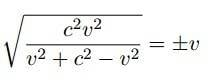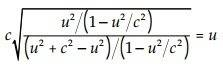# Simplifying square root

• lioric

#### lioricHow is it equal to v in the end?
I'm sorry for asking such questions. But I'm just trying to understand

Because addition is associative and commutative and ##c^2≠0##. For short: just calculate the left hand side.

Can you simplify the denominator?

Can you simplify the denominator?
Dear god I must be blind
v^2-v^2 = 0
c^2 and c^2 cancels
And all that's left is a rooted v^2 which cancels itself

But look at thisThis is how the same solution is illustrated in another book.
(1-u^2/c^2) cancels each other
The u^2 subtracts each other in the denominator like in the previous sollution
Which leaves a rooted u^2 / c^2
How does that simplify?

Which leaves a rooted u^2 / c^2
How does that simplify?
Look to the left of the square root sign...

Look to the left of the square root sign...
I can see the c root What does it mean?

I can see the c root What does it mean?
No, it's c times the root.

No, it's c times the root.
So are you saying that the large root will cancel the squares of u^2 / c^2 making it like u / c * c/1
and c and c cancels?

So are you saying that the large root will cancel the squares of u^2 / c^2 making it like u / c * c/1
and c and c cancels?
Yes. Note that the author there takes only the positive root, while in the OP the two roots are kept.

Yes. Note that the author there takes only the positive root, while in the OP the two roots are kept.
Thank you very much

View attachment 96291
How is it equal to v in the end?
I'm sorry for asking such questions. But I'm just trying to understand
This is actually incorrect. On the right side it should be |v|, not ##\pm v##. In other words, the square root evaluates to a single nonnegative number, not two numbers.